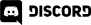# Home > Programming >

26. November 2012

Collisions are one of those things almost every game deal with eventually.  This is going to be a multi-part recipe on collision detection.  This part specifically explains what an axis aligned bounding box actually is, as well as how to detect if two such bounding boxes have collided.

Let's start straight away with some code, then we will discuss the details a bit more later on.  This example simply animates one bounded sprite until it collides with another.  Once an intersection is detected, the positions are reset and the whole things starts all over again.

## Just the Math

Creating the bounding box:

if(createjs.Bitmap.prototype.getBoundingRect == null){

createjs.Bitmap.prototype.getBoundingRect = function(){

return new createjs.Rectangle(

this.x - this.image.width/2,

this.y - this.image.height/2,

this.image.width,

this.image.height);

}

}

Checking if two bounding boxes intersect:

if(createjs.Rectangle.prototype.intersects == null){

createjs.Rectangle.prototype.intersects = function(rect){

return (this.x <= rect.x + rect.width &&

rect.x <= this.x + this.width &&

this.y <= rect.y + rect.height &&

rect.y <= this.y + this.height);

}

}

## Description

The creation of a bounding box is pretty simple.  In this case, our sprite's pivot point is at it's centre, so our top left corner is half the height and width away from our position.  If you are unfamiliar with JavaScript, some of this code might look a bit confusing.  Basically what we are doing is adding a method to the "class" Bitmap named getBoundingRect, but first we want to make sure it hasn't already been added.  We perform the same process when we add the intersects method to the Rectangle "class".  Obviously if you aren't using JavaScript or your language/library of choice includes such functionality already, you can skip that part of the process.

The intersects method works using this simple logic.

Given two rectangles, say R1 and R2, they intersect if:

• The left side of R1 is NOT to the right of the right side of R2
• The left side of R2 is NOT to the right of the right side of R1
• The top of R1 is NOT below the bottom of R2
• The top of R2 is NOT below the bottom of R1

If all of those conditions are true, the two rectangles intersect.  The concept is extremely simple, but making sense of the words isn't always the case.  So here is an image illustrating how it works:Now earlier on I used the words axis-aligned bounding boxes.  What do I mean by axis-aligned?  Well don't worry, it's a lot less scary than it sounds.  It simply means that the two sides of the bounding box are aligned with both the X and Y axis.  Again, Ill use a graphic to illustrate.Both the top and bottom bounding boxes are axis aligned, in that the sides of the bounding box are parallel to the X and Y axis.  Using axis aligned bounding boxes make the collision test a great deal faster, but comes at a price.  As you see from the middle image, you cannot simply rotate the bounding box if you rotate the sprite, instead as you can see from the bottom example, you resize the bounding box to encompass the new dimensions of the rotated ( or scaled ) sprite.

How do you do this?  That we will discuss in the next section!

## Complete Code

A word of warning, much of the code below is a) extending the EaselJS to have bounding box support b) to actually (hackishly I admit) render the bounding box on screen.  Do not let the size deter you, the process is actually quite simple and obviously you don't need to draw the bounding box most of the time.

<!DOCTYPE html>

<html>

<script src="http://code.createjs.com/easeljs-0.5.0.min.js"></script>

<script>

var jetSprite,jetSprite2;

var stage;

if(createjs.Rectangle.prototype.intersects == null){

createjs.Rectangle.prototype.intersects = function(rect){

return (this.x <= rect.x + rect.width &&

rect.x <= this.x + this.width &&

this.y <= rect.y + rect.height &&

rect.y <= this.y + this.height);

}

}

if(createjs.Bitmap.prototype.getBoundingRect == null){

createjs.Bitmap.prototype.getBoundingRect = function(){

return new createjs.Rectangle(

this.x - this.image.width/2,

this.y - this.image.height/2,

this.image.width,

this.image.height);

}

}

function demo(){

stage = new createjs.Stage("theCanvas");

jetSprite = new createjs.Bitmap("jetsprite.small.png");

jetSprite.regX = 30; // Half image width

jetSprite.regY = 40; // Half image height

jetSprite.y = 200;

jetSprite.x = 100;

// Add a Image load handler, as image.width and image.height are meaningless until

// load is done and DOMContentLoaded doesn't seem to take into account dynamic images

// Draw the bounding rect and create a new Shape with it

var g = new createjs.Graphics();

g.setStrokeStyle(1);

g.beginStroke(createjs.Graphics.getRGB(255,255,255));

var bb = jetSprite.getBoundingRect();

g.drawRect(bb.x,bb.y,bb.width,bb.height);

jetSprite.boundingBox = new createjs.Shape(g);

}

jetSprite2 = new createjs.Bitmap("jetsprite.small.png");

jetSprite2.regX = 30; // Half image width

jetSprite2.regY = 40; // Half image height

jetSprite2.y = 200;

jetSprite2.x = 300;

var g = new createjs.Graphics();

g.setStrokeStyle(1);

g.beginStroke(createjs.Graphics.getRGB(255,255,255));

var bb = jetSprite2.getBoundingRect();

g.drawRect(bb.x,bb.y,bb.width,bb.height);

jetSprite2.boundingBox = new createjs.Shape(g);

}

//And go...

stage.update();

// onFrame will be called each "tick". Default is 50ms, or 20FPS

}

function onFrame(elapsedTime) {

// Convert from milliseconds to fraction of a second

var delta = elapsedTime /1000;

// Move sprite and bounding box shape to the right

jetSprite.boundingBox.x++;

jetSprite.x++;

if(jetSprite.getBoundingRect().intersects(jetSprite2.getBoundingRect())){

// If a collision occurs, reset position and start all over again

jetSprite.x = 100;

jetSprite.boundingBox.x = 0;

}

stage.update();

}

</script>

<body>

<canvaswidth=400 height=400 id="theCanvas" style="background-color:black"/>

</body>

</html>